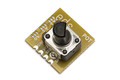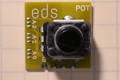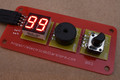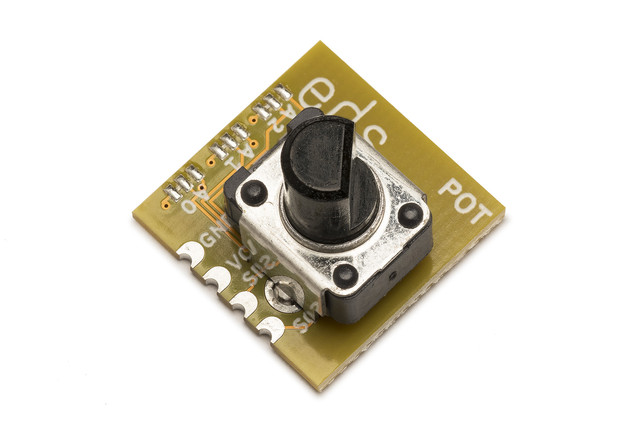# Electric Dollar Store

••••• ## I²C Digital Potentiometer

• Twist potentiometer rotates 270°
• 200 Hz update rate
• Selectable scale from 0-1 to 0-255
• standard 18x18mm module with I²C and power
• Use with knob2

• \$1.80

POT is a high-quality twist potentiometer coupled with an I²C controller which reports the instantaneous rotary position.

The I²C protocol is straightforward. The device has 255 locations. Reading from location N gives the rotary position scaled to the range (0..N). So for example reading from 100 gives a position value between 0 (fully counter-clockwise) to 100 (fully clockwise). In addition, reading from location 0 gives the knob rotation as a 16-bit unsigned value.

```import sys
import struct
import time

from i2cdriver import I2CDriver, EDS

if __name__ == '__main__':
i2 = I2CDriver(sys.argv)

d = EDS.Pot(i2)

while 1:
percentage = d.rd(100)
r = d.raw()

print("%3d/100   raw=%3d" % (percentage, r))
time.sleep(.05)
```
```#include <Wire.h>

{
Wire.beginTransmission(0x28);
Wire.write(scale);
Wire.endTransmission(false);
Wire.requestFrom(0x28, 1);
}

void setup() {
Serial.begin(115200);
Wire.begin();
}

void loop() {
delay(20);
}
```
```from machine import I2C
import struct
import time

class Pot:
""" POT is an analog knob potentiometer """
def __init__(self, i2, a = 0x28):
self.i2 = i2
self.a = a

def raw(self):
"""
Return the current knob rotation as a 16-bit integer.
"""

def rd(self, r):
"""
Return the current knob rotation, scaled to the range 0 .. r
inclusive. For example rd(100) returns a value in the range 0 to 100.
"""
 Default I²C address `0x28` (`0b0101000`) Current consumption (typ.) 5 mA Vcc 2.2 - 3.6 V